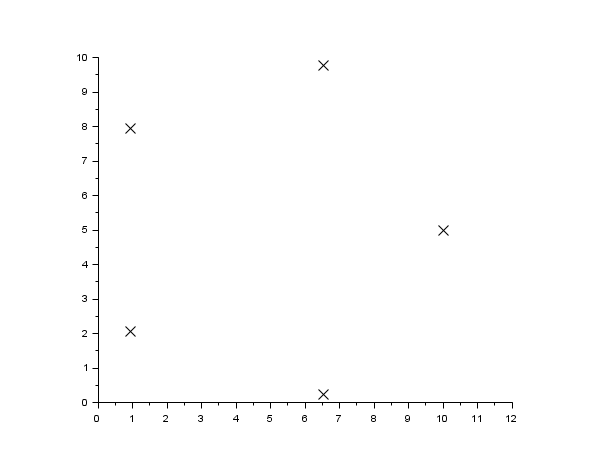Scilab Home page | Wiki | Bug tracker | Forge | Mailing list archives | ATOMS | File exchange
Scilab 6.0.1
Change language to: English - Français - Português - 日本語 -

Please note that the recommended version of Scilab is 6.0.2. This page might be outdated.
See the recommended documentation of this function

Справка Scilab >> Графики > polygon > xpoly

xpoly

draw a polyline or a polygon

Syntax

xpoly(xv,yv [,dtype [,close]])

Arguments

xv,yv

matrices of the same size (points of the polyline).

dtype

string (drawing style). default value is "lines".

close

integer. If close=1, the polyline is closed; default value is 0.

Description

xpoly draws a single polyline described by the vectors of coordinates xv and yv. If xv and yv are matrices they are considered as vectors by concatenating their columns. dtype can be "lines" for using the current line style or "marks" for using the current mark to draw the polyline.

Examples

x=sin(2*%pi*(0:4)/5);
y=cos(2*%pi*(0:4)/5);

plot2d(0,0,-1,"010"," ",[-2,-2,2,2])
xpoly(x,y,"lines") // by default closed
e=gce(); // get the current entity (the last created: here the polyline)
set(e,"foreground",5);

e.closed = 'off' // the polyline is now open// With marks instead of lines
plot2d(0,0,1,rect=[0,0,12,10], frameflag=3)
t=[0:4]*2*%pi/5;
x=5+5*cos(t);
y=5+5*sin(t);
xpoly(x,y,"marks")
e=gce();
set(e,"mark_style",2);